# Kerala Syllabus 6th Standard Maths Solutions Chapter 11 Statistics

## Kerala State Syllabus 6th Standard Maths Solutions Chapter 11 Statistics

### Statistics Text Book Questions and Answers

Bar graphs Textbook Page No. 165

Remember how we drew bar graphs to show numerical information? See the bar graph below.
It shows the amount of money each class in a school donated to the Snehasparsham medical aid fund.• What is the total amount donated?
• Which class gave the most?
• And the least?

What other things should we get from this table?
The other things we get from the data given are What is the average amount denoted? and
How many classes gave the funds?

Explanation:
From the data given above, the other things we get:
What is the average amount denoted?
How many classes gave the funds?The table below shows the number of children in each class in a school.Let’s draw a bar graph of this.
The height of each bar should be according to the number of children. If we take it as 1 centimetre per child, what would be the heights?
So, what length per child would be a convenient scale?
What other things should we decide to draw the graph?

• The width of a bar
• Distance between barsNow draw a graph in your notebook.
We get the number of children in each class from this graph and we get the amount each class donated from the first graph.

For example, in class VA, there are 25 children and they donated 600 rupees. So how much did each child of this class give on average?
Calculate the average amount for other classes also.

• Which class has the highest average?
VIIA class has the highest average.

Explanation:
Number of students of VA = 25.
Total amount donated by VA = 600 rupees.
Amount denoted by each student = Total amount donated by VA ÷ Number of students of VA
= 600 ÷ 25
= 24.
Number of students of VB = 30.
Total amount donated by VB = Amount denoted by each student × Number of students of VB
= 30 × 24
= 720.
Number of students of VIA = 30.
Total amount donated by VIA = Amount denoted by each student × Number of students of VIA
= 30 × 24
= 720.
Number of students of VIB = 20.
Total amount donated by VIB = Amount denoted by each student × Number of students of VIB
= 20 × 24
= 480.
Number of students of VIIA = 40.
Total amount donated by VIIA = Amount denoted by each student × Number of students of VIIA
= 40 × 24
= 960.
Number of students of VIIB = 35.
Total amount donated by VIIB = Amount denoted by each student × Number of students of VIIB
= 35 × 24
= 840.
Total amount donated = 600 + 720 + 480 + 960 + 840
= 3600.
Total number of students = 25 + 30 + 30 + 20 + 40 + 35
= 180.
Average amount donated = Total amount donated ÷ Total number of students
= 3600 ÷ 180
= 20.
Average of VA = 600 ÷ 20 = 30.
Average of VB = 720 ÷ 20 = 36.
Average of VIA = 720 ÷ 20 = 36.
Average of VIB = 480 ÷ 20 = 24.
Average of VIIA = 960 ÷ 20 = 48.
Average of VIIB = 840 ÷ 20 = 42.

• And the lowest?
VIB Class the lowest.

Explanation:
Number of students of VA = 25.
Total amount donated by VA = 600 rupees.
Amount denoted by each student = Total amount donated by VA ÷ Number of students of VA
= 600 ÷ 25
= 24.
Number of students of VB = 30.
Total amount donated by VB = Amount denoted by each student × Number of students of VB
= 30 × 24
= 720.
Number of students of VIA = 30.
Total amount donated by VIA = Amount denoted by each student × Number of students of VIA
= 30 × 24
= 720.
Number of students of VIB = 20.
Total amount donated by VIB = Amount denoted by each student × Number of students of VIB
= 20 × 24
= 480.
Number of students of VIIA = 40.
Total amount donated by VIIA = Amount denoted by each student × Number of students of VIIA
= 40 × 24
= 960.
Number of students of VIIB = 35.
Total amount donated by VIIB = Amount denoted by each student × Number of students of VIIB
= 35 × 24
= 840.
Total amount donated = 600 + 720 + 480 + 960 + 840
= 3600.
Total number of students = 25 + 30 + 30 + 20 + 40 + 35
= 180.
Average amount donated = Total amount donated ÷ Total number of students
= 3600 ÷ 180
= 20.
Average of VA = 600 ÷ 20 = 30.
Average of VB = 720 ÷ 20 = 36.
Average of VIA = 720 ÷ 20 = 36.
Average of VIB = 480 ÷ 20 = 24.
Average of VIIA = 960 ÷ 20 = 48.
Average of VIIB = 840 ÷ 20 = 42.

In class VI, 20 children got A grade, 50 got B grade, 20 got C grade, 15 got D grade and 5 got E grade. Draw a bar graph showing this.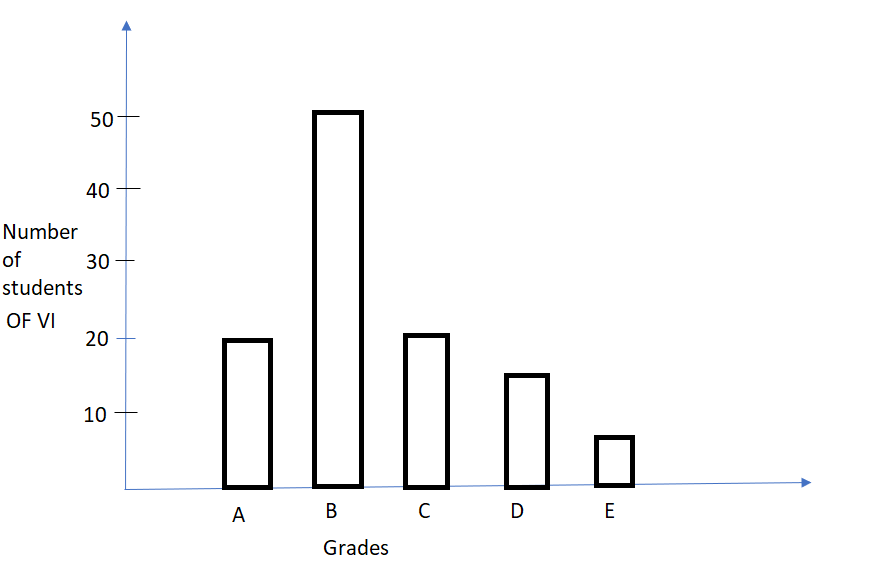Explanation:
Number of students of VI – Y-axis representation.
Types of Grades – X- axis representation.Double bar

The bar graph below shows the number of boys and girls present in class 5 of a school, from 1st to 5th of June.Complete the table, based on this.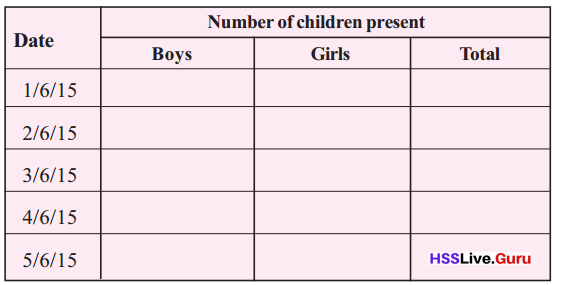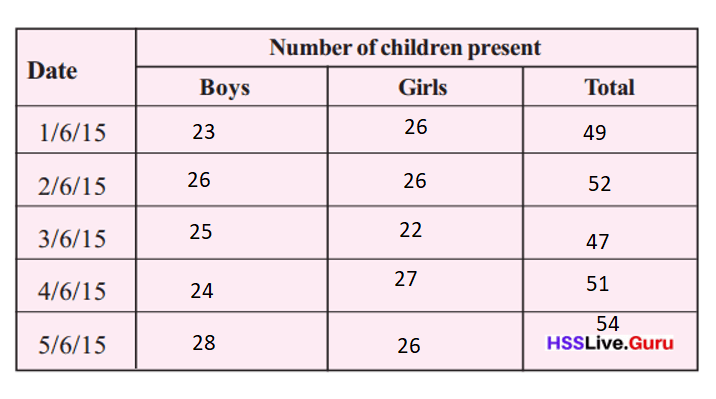Explanation:
1/6/15 – Boys = 23    Girls = 26     Total = 23 + 26 = 49.
2/6/15 – Boys = 26   Girls = 26      Total = 26 + 26 = 52.
3/6/15 – Boys = 25   Girls =  22     Total = 25 + 22 = 47.
4/6/15 – Boys = 24    Girls = 27      Total = 24 + 27 = 51.
5/6/15 – Boys = 28    Girls = 26      Total = 28 + 26 = 54.

• On which day was the least number of children present?
On 3/6/15 was the least number of children present.

Explanation:
1/6/15 – Boys = 23    Girls = 26     Total = 23 + 26 = 49.
2/6/15 – Boys = 26   Girls = 26      Total = 26 + 26 = 52.
3/6/15 – Boys = 25   Girls =  22     Total = 25 + 22 = 47.
4/6/15 – Boys = 24    Girls = 27      Total = 24 + 27 = 51.
5/6/15 – Boys = 28    Girls = 26      Total = 28 + 26 = 54.

• On which day was the most number of boys present? And the least?
On 5/6/15 was the most number of boys present and 1/6/15 the least.

Explanation:
1/6/15 – Boys = 23    Girls = 26     Total = 23 + 26 = 49.
2/6/15 – Boys = 26   Girls = 26      Total = 26 + 26 = 52.
3/6/15 – Boys = 25   Girls =  22     Total = 25 + 22 = 47.
4/6/15 – Boys = 24    Girls = 27      Total = 24 + 27 = 51.
5/6/15 – Boys = 28    Girls = 26      Total = 28 + 26 = 54.
Most boys – 5/6/15.
Least boys – 1/6/15

On 4/6/15 was the most number of girls present and 3/6/15 the least.

Explanation:
1/6/15 – Boys = 23    Girls = 26     Total = 23 + 26 = 49.
2/6/15 – Boys = 26   Girls = 26      Total = 26 + 26 = 52.
3/6/15 – Boys = 25   Girls =  22     Total = 25 + 22 = 47.
4/6/15 – Boys = 24    Girls = 27      Total = 24 + 27 = 51.
5/6/15 – Boys = 28    Girls = 26      Total = 28 + 26 = 54.
Most girls – 4/6/15.
Least girls – 3/6/15

• On which day was the difference in number between boys and girls the most?
1/6/15, 3/6/15 and 4/6/15 these days the difference in number between boys and girls the most.

Explanation:
1/6/15 – Boys = 23    Girls = 26     Total = 23 + 26 = 49.
2/6/15 – Boys = 26   Girls = 26      Total = 26 + 26 = 52.
3/6/15 – Boys = 25   Girls =  22     Total = 25 + 22 = 47.
4/6/15 – Boys = 24    Girls = 27      Total = 24 + 27 = 51.
5/6/15 – Boys = 28    Girls = 26      Total = 28 + 26 = 54.
Total boys and girls = 49 + 52 + 47 + 51 + 54 = 253.
Difference on 1/6/15:
26 – 23 = 3.
Difference on 2/6/15:
26 – 26 = 0.
Difference on 3/6/15:
25 – 22 = 3.
Difference on 4/6/15:
27 – 24 = 3.
Difference on 5/6/15:
28 – 26 = 2.
There is no specific date for the difference in number between boys and girls the most. Major difference of 3 is on three days of 3.

100 grams of rice is taken per child for lunch. How much rice was used each day during this week?
Total quantity of rice children nedded for a week = 1,77,100 grams.

Explanation:
Number of grams of rice is taken per child for lunch = 100.
Number of days in a week = 7.
Total number of boys and girls = 49 + 52 + 47 + 51 + 54 = 253.
Number of grams each children eats for lunch = Number of grams of rice is taken per child for lunch × Total number of boys and girls
= 100 × 253
= 25,300 grams.
Total quantity of rice children nedded for a week = Number of grams each children eats for lunch × Number of days in a week
= 25,300 × 7
= 1,77,100 grams.

Textbook Page No. 168

Question 1.
The table shows the number of notebooks sold at the school store during six months.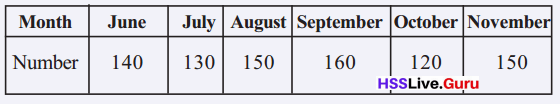Draw a bar graph of this.Explanation:
June – 140 note books.
July – 130 note books.
August – 150 note books.
September – 160 note books.
October – 120 note books.
November – 150 note books.

Question 2.
The table shows the various expenses of George’s family for the last month.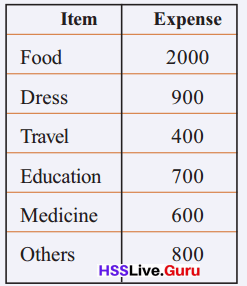Draw a bar graphs of this. Write down some facts we get from the graph.Explanation:
Less expense the family invests on Travelling.
Most of their expenses is consumption of food.

Question 3.
The table below shows the amount of electricity used at Soumya’s home during last year.i. How many units were used in all last year?
Total number of units used in the last year = 2100 KW.

Explanation:
Number of units used in:
Month = January, February = 340 KW.
Month = March, April = 440 KW.
Month = May, June = 410 KW.
Month = July, August = 290 KW.
Month = September, October = 300 KW.
Month = November, December = 320 KW.
Total number of units used in the last year = 340 KW + 440 KW + 410 KW + 290 KW + 300 KW + 320 KW = 2100 KW.

ii. What is the average use every two months?
Average use of January, February = 340 KW ÷ 2 = 170 KW.
Average use of  March, April = 440 KW ÷ 2 = 220 KW.
Average use of May, June = 410 KW ÷ 2 = 205 KW.
Average use of July, August = 290 KW ÷ 2 = 145 KW.
Average use of September, October = 300 KW ÷ 2 = 150 KW.
Average use of November, December = 320 KW ÷ 2 = 160 KW.

Explanation:
Number of units used in:
Month = January, February = 340 KW.
Average use of January, February = 340 KW ÷ 2 = 170 KW.
Month = March, April = 440 KW.
Average use of  March, April = 440 KW ÷ 2 = 220 KW.
Month = May, June = 410 KW.
Average use of May, June = 410 KW ÷ 2 = 205 KW.
Month = July, August = 290 KW.
Average use of July, August = 290 KW ÷ 2 = 145 KW.
Month = September, October = 300 KW.
Average use of September, October = 300 KW ÷ 2 = 150 KW.
Month = November, December = 320 KW.
Average use of November, December = 320 KW ÷ 2 = 160 KW.

iii. During which two months was the use closest to the average?
Average use of January, February of 170 KW is the use closest to the average.

Explanation:
Total number of units used in the last year = 2100 KW.
Average use in last year = 2100 KW ÷ 12 = 175.
Average use of January, February = 340 KW ÷ 2 = 170 KW.
Average use of  March, April = 440 KW ÷ 2 = 220 KW.
Average use of May, June = 410 KW ÷ 2 = 205 KW.
Average use of July, August = 290 KW ÷ 2 = 145 KW.
Average use of September, October = 300 KW ÷ 2 = 150 KW.
Average use of November, December = 320 KW ÷ 2 = 160 KW.Question 4.
The bar graph shows the percent of those who voted in some wards in a panchayath election.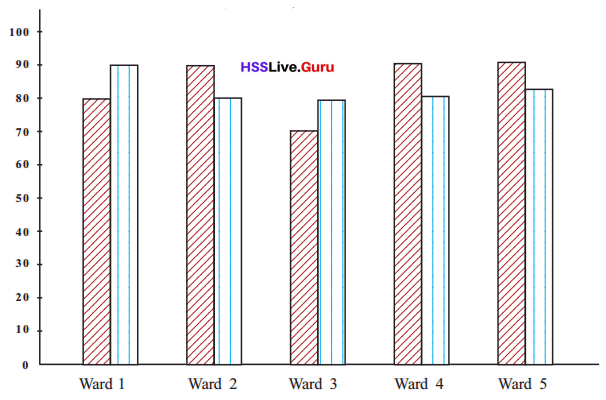The table shows the total number of voters.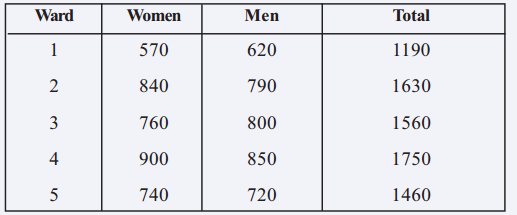Calculate the actual number of men and women who voted in each ward.
Ward 1: Actual number of total = 558 + 456 = 1,014.
Ward 2: Actual number of total = 632 + 756 = 1,388.
Ward 3: Actual number of total = 640 + 532 = 1,172.
Ward 4: Actual number of total = 680 + 810 = 1,490.
Ward 5: Actual number of total =576 + 666 = 1,242.

Explanation:
Ward 1:
Number of men = 620 × 90%
= 620 × 90 ÷ 100
= 62 × 9
= 558.
Number of Women = 570 × 80%
= 570 × 80 ÷ 100
= 57 × 8
= 456.
Actual number of total = 558 + 456 = 1,014.
Ward 2:
Number of men = 790 × 80%
= 790 × 80 ÷ 100
= 79 × 8
= 632.
Number of Women = 840 × 90%
= 840 × 90 ÷ 100
= 84 × 9
= 756.
Actual number of total = 632 + 756 = 1,388.
Ward 3:
Number of men = 800 × 80%
= 800 × 80 ÷ 100
= 80 × 8
= 640.
Number of Women = 760 × 70%
= 760 × 70 ÷ 100
= 76 × 7
= 532.
Actual number of total = 640 + 532 = 1,172.
Ward 4:
Number of men = 850 × 80%
= 850 × 80 ÷ 100
= 85 × 8
= 680.
Number of Women = 900 × 90%
= 900 × 90 ÷ 100
= 90 × 9
= 810.
Actual number of total = 680 + 810 = 1,490.
Ward 5:
Number of men = 720 × 80%
= 720 × 80 ÷ 100
= 72 × 8
= 576.
Number of Women = 740 × 90%
= 740 × 90 ÷ 100
= 74 × 9
= 666.
Actual number of total =576 + 666 = 1,242.

error: Content is protected !!